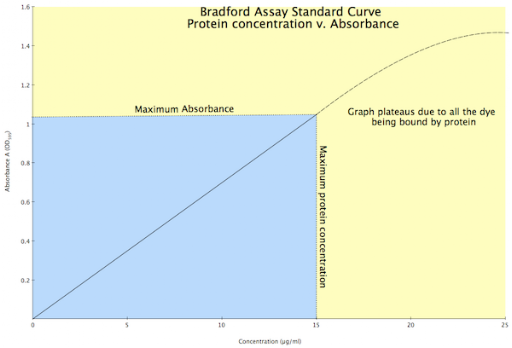## Wednesday, 20 October 2010

### Why can't I extrapolate the Bradford Assay graph if the Beer-Lambert Law applies?

If you are struggling with 'science maths' then have a look at Maths4Biosciences - there is also a course on Beer-Lambert Law and Spectrophotometry.

If you would like to test your skills working with the Beer-Lambert Law then you might like to look at the Spectrophotometry tests at: Maths4Biosciences.com.

One question I have been asked a number of times after the feedback on a practical is if the Beer-Lambert Law, A = ε . c . l, gives a straight line, then why can't I extrapolate the data to find the answer for one of my unknown proteins?

The Bradford Assay is an indirect (or colorimetric) method for estimating protein concentrations. The assay is actually based on a shift in the absorbance of a dye, Coomassie Brilliant Blue G-250, from 465 nm to 595 nm when it binds a protein under acidic conditions. That is, the Bradford assay is really measuring the amount of bound dye and NOT the amount of protein present (although the two are connected until the dye is all used). As the dye is limiting (i.e. you only put in a set amount), then you can reach a point when all the dye is bound, and no matter how much more protein you put in you will not see a change in absorbance. That is, you can increase the protein level to a point where available dye becomes exhausted so the graph would plateau.Standard Curve for a Bradford Protein Assay

Any value that falls in the yellow area of the graph (i.e. a concentration of more than 15 µg/ml, or an absorbance greater than 1.02) cannot be used as the graph is no longer linear and is starting to plateau. Any absorbance that give a protein concentration below 15 µg/ml can be used as this is in the linear portion of the graph (i.e. blue area), and the Beer-Lambert Law applies as the dye is not limiting.

In the experiment you are working in the linear portion of the graph above (the blue area) and so the line is straight, i.e. it hasn't plateaued. Therefore we can say that between 0 and x µg/ml (or 15 µg/ml in the above) we have a certain absorption range, say 0 to y (where y is about 1.01 in the above figure). However, once we get above x µg/ml, or above the maximum absorbance y, we cannot say whether or not the graph has plateaued, so all we can say is the value is greater than x µg/ml. This may seem somewhat limiting (pardon the pun), but the Bradford assay can in fact detect protein concentrations over a 10-fold concentration range.

By the way, if we were measuring the protein directly, say at 280 nm, then we could apply the Beer-Lambert Law, in fact, if we knew extinction coefficient we wouldn't even have to do a standard curve.

If you are struggling with 'science maths' then have a look at Maths4Biosciences - there is also a course on Beer-Lambert Law and Spectrophotometry.

1.